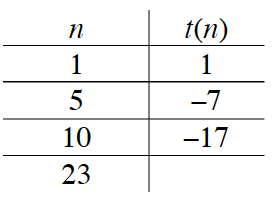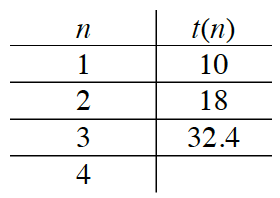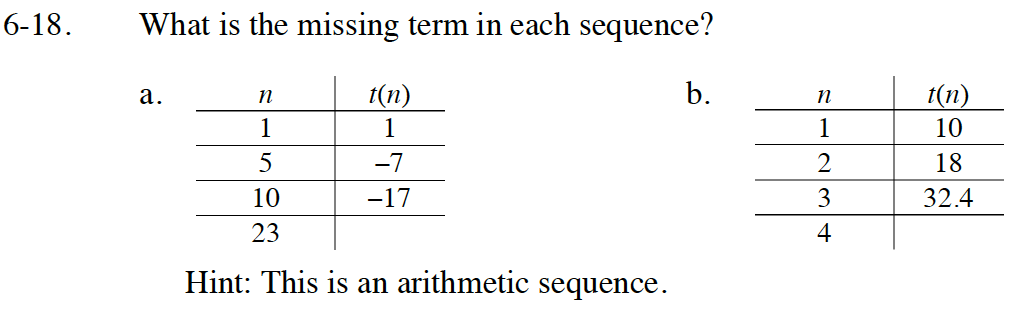Home > GB8I > Chapter cc46 > Lesson cc46.1.2 > Problem6-18

6-18.
1. What is the missing term in each sequence? Homework Help ✎

2.  a.Hint: This is an arithmetic sequence. b.Review the Math Notes box in section 5.3.2

Note that there are terms missing in the table.
a1 = 1 while a5 = −7.
What is the common difference?

Review the Math Notes box in section 5.3.2
Is this Arithmetic or Geometric?

This is a Geometric sequence.

$\frac{a_{2}}{a_{1}}=\frac{18}{10}=1.8$

$\frac{a_{3}}{a_{2}}=\frac{32.4}{18}=1.8$

So r = 1.8

a4 = a3 · 1.8

a4 = 32.4 · 1.8

a4 = 58.32